## Pages

### FIGURE OUT PUZZLES - matchstick puzzles (01)Question 1
Move three matchsticks to correct the equation.
There's more than two solutions.

## View answer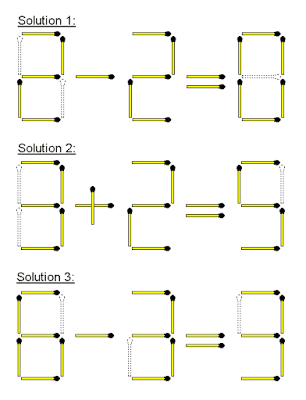Question 2
Move two matchsticks to make the equation true.
*More than one solution

## View answer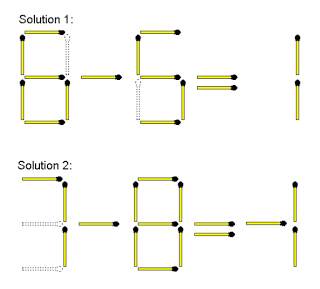Question  3
Move one matchstick to equal the number two

## View answerQuestion  4

## View answerQuestion  5
Move only 1 matchstick to create 2 squares

## View answer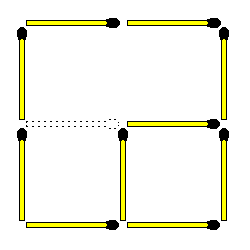Question  6
Move two matchsticks to make the equation true

## View answer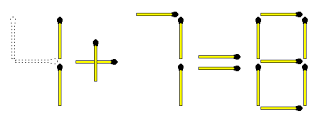Question  7
Add two matchsticks to make the equation true

## View answer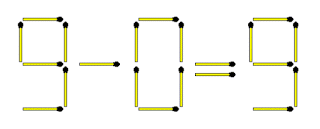Question  8
Move only 2 matchsticks to create 6 squares and 4 rectangles. The following rules apply:
.
1. Squares must be equal in size
2. Rectangles must be equal in size
3. Not all squares or rectangles have to be connected

## View answer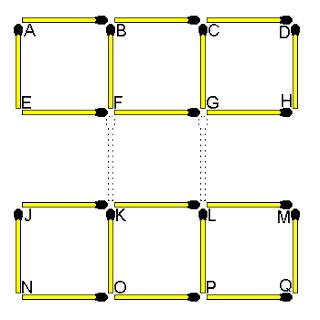Question 9
Take away two matchsticks to make the equation true

## View answerQuestion 10
Move 3 matchsticks to create 9 squares. The squares must be equal in size

## View answerQuestion 11
What is the relationship or link between the 4 figures below?

## View answer

Remove the same 2 matchsticks from each figure and turn your head to the right. It forms the letters V W X Y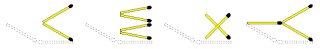Question 12
Can you decipher the hidden word in this figure by linking row 1 with row 2

## View answer

By flipping row 1 and row 2 upside down and merging them togetherQuestion  13
Move two matchsticks to make the equation true.
*More than one solution

## View answer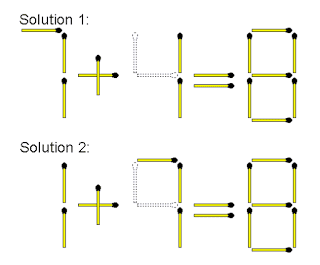Question  14
Move two matchsticks to make this equation correct.
*more than two solutions

## View answerQuestion  15
Take away only 1 matchstick to leave 4 equal squares. All the squares must be connected and all matchsticks must form part of a square.

## View answerQuestion  16

## View answer

C is the odd one out. Adding 1 matchstick to any other layout forms a capital letter.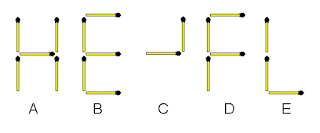Question  17
Move three matchsticks to make the equation true

## View answer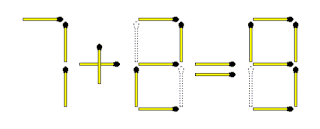Question  18
Take away two matchsticks to make the equation true

## View answerQuestion 19
How would you show that this equation is correct by just adding only one matchstick

## View answerQuestion 20
Move one matchstick to make this equation true

## View answerShare this article with your friends.